# Zeros of Polynomial Functions

In the previous article, where we introduce polynomials, a brief introduction to the roots of quadratic equations were discussed with some examples. Now you are going to learn the zeros of polynomial in more detail.

The idea of zero is that to find those x values for which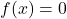and it is the x-intercepts in case of quadratic equations which is also a polynomial of degree 2.

### Rational Zero Theorem

As we mentioned earlier, the zeros or roots of a polynomial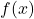is nothing but those values of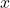for which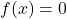.

If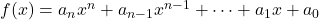is a polynomial with integer coefficients, then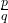is the rational zero of the polynomialwhere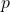is the factor of constant term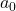and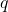is the factor of leading coefficient.

This is could be understood with the help of an example.

Example #1

Find the rational zeros of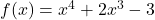.

Solution:

The constant term is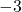and its factors are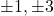.

Similarly, the leading coefficient iswhose factors are.

Therefore,

Rational Zeros =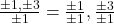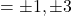There are total 8 roots for this polynomial equation. The actual roots are.

### How To Use The Rational Zero Theorem?

We can use the rational zeros to find the real zero of the polynomial. Note that the polynomial is divided by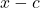such that.

Example #2

Find the real root of the polynomial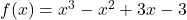from its rational zeros.

Solution:

The constant term is -3 so the factors of constant terms are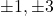.

The leading coefficient term is 1 and the factors of leading coefficient are.

Rational \hspace{2 mm} zeros = \frac{\pm1, \pm3}{\pm1}
= \frac{\pm1}{\pm1}, \frac{\pm3}{\pm1}
=\pm1, \pm3

Now we test each of the root to find the real root of the polynomial. For this we have to use synthetic division. If you are not familiar with the synthetic division, read the previous article.

3 : 1 -1 3 -3
3 6  27   ( This solution is not suitable because remainder is 24. The remainder should be 0).
1  2 9  24

1 : 1 -1 3 -3
1 0  3   ( The real root of the polynomial is 1 ).
1  0 3  0


After performing a synthetic division we are able to find the real root of the polynomial. You can also plot the graph of the polynomial and then look for root which is x-intercept.

### Solving Polynomial Equations

The rational zeros of polynomials are very helpful when the degrees of polynomial equations are higher. Consider the following example.

Example #3

Find the solution to the polynomial equation: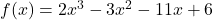.

Solution:

To find solution we must find all the rational roots.

The factors of constant 6 are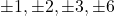and similarly, the factors of leading coefficient is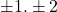.

The rational coefficients are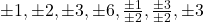.

Synthetic Division

Now we can do synthetic division to find the solution.

1 : 2 -3 -11 6
2  5 -6  ( 1 is a root of polynomial f(x).
2  5 -6  0

Now we can write the equation as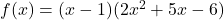and remainder is 0.

Example #4

Find the rational root of the following polynomial: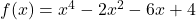.

Solution:

The factors of the constant terms are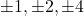.

The leading coefficient has following factors :.

The rational roots are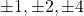.

Synthetic division

To find the factors we now have to use the synthetic division. First we are going test1: 1 -2 -6 4
1  1 -5  ( This has a remainder of -1. We need to have remainder of 0 in the last cell.)
1  1 -5 -1

2: 1 -2 -6 4
2  0 -12  ( This has a remainder of -8. We need to have remainder of 0 in the last cell.)
1  0 -6 -8

-2: 1 -2 -6 4
-2  8 -4  ( This has a remainder of 0. )
1 -4  2 0

Therefore, the root of the equation isand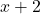is a factor.

 f(x) = (x + 2)(x^2 - 4x + 2)

We can use the quadratic formula to solve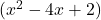and find rest of the roots.

x = \frac{-b \pm \sqrt{b^2 - 4ac}}{2a} \\ \\
= \frac{4 \pm 2\sqrt{2}}{2} \\ \\
x = 2 + \sqrt{2}  \hspace{2mm} and \hspace{2mm} x = 2 - \sqrt{2}

### Fundamental Theorem Of Algebra

The fundamental theorem of algebra states that a polynomialwith a degree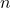has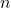roots. To understand this consider the following graph.

Ifis a polynomial with degreewhere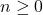, then the equation has one complex root.

Example #5

From our earlier example,

We have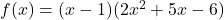we can factor the second degree polynomial using quadratic formula.

 x = \frac{-5 \pm \sqrt{25 - 48}}{4}
= \frac{-5 \pm i\sqrt{23}}{4}


The roots are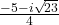and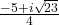. The complex conjugate is also a root.

### Linear Factorization

The linear factorization is states that for a polynomialwhere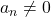, then the polynomial can be written as product of linear factors, called the linear factorization.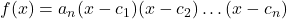where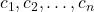are complex numbers.

Example #6

Find the factors of the polynomial: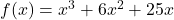.

Solution:

The factors are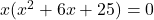Using the quadratic formula,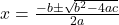x = \frac{-6 \pm \sqrt{36-100}}{2}\\ \\
= \frac{-6 \pm \sqrt{-64}}{2}\\ \\
= \frac{-6 \pm i8}{2} \\


Therefore, the roots are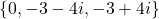We can write the polynomial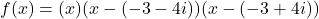This means that roots are rational , irrational and complex or imaginary numbers. In the next section, we discuss a method to find the real roots for polynomial functions.

### Rules of Signs

The rules of signs is also called the Descartes’s rules of signs. In a polynomial with degree, there are at mostreal roots, the rule of signs provide specific information about the number of real roots in a polynomial equation.

If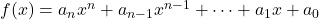is a polynomial with degreewith real coefficients, then

number of positive real roots are:

1. same as the number of sign changes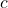in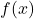or
2. less than number of sign changeby a positive even integer.
3. If there is only one sign change in, then there is only one real root.

number of negative real roots are:

1. same as the number of sign changes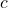in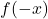or
2. less than number of sign changeby a positive even integer.
3. If there is only one sign change in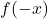, then there is exactly one real root.

Example #7

Find the number of real roots in the following polynomial:

f(x) = x^3-7x^2+41x-87

Solution:

First we will find the number of sign changes in the given polynomial function.

The above equation , there are 3 signs change. Therefore, there are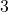positive real roots of.

Or since,is positive, we have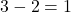positive real root.

We can find the zeros of the polynomial to confirm this. The factors of the polynomials are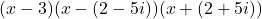.

How Rules Of Signs Are Helpful In Solving Polynomials?

The rules of signs helps us to eliminate the unwanted roots from all the rational roots. To illustrate this, we use an example.

Example #8

Find the real roots of the following polynomial: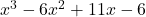.

Solution:

The number of sign change in this polynomial is 3, therefore, real roots areor.

Let us find the rational roots of this equation.

Factors of the constant terms is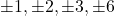.

Factors of the leading coefficient is.

Rational \hspace{2mm}roots =  \frac{\pm 1, \pm 2 ,\pm 3, \pm 6}{\pm 1} = \pm 1, \pm 2 ,\pm 3, \pm 6

The rules of sign inform us that there is no negative real roots in this equation. Therefore, we can eliminate all the negative values. The remaining rational roots are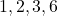.

Test With Synthetic Division

1: 1 -6 11 -6
1 -5  6 ( Since, the remainder is zero, 1 is a positive real root of.
1 -5  6  0

3: 1 -6 11 -6
3 -9  6 ( Since, the remainder is zero, 3 is a positive real root of.
1 -3  2  0

2: 1 -6 11 -6
2 -8  6 ( Since, the remainder is zero, 2 is a positive real root of.
1 -4  3  0

There are three real roots as per rules of signs.

### Summary

In this article, you have learned how to find the rational roots, and under special circumstance such as, there are complex or imaginary roots, so we can find these roots with the linear factorization and rules of signs technique.